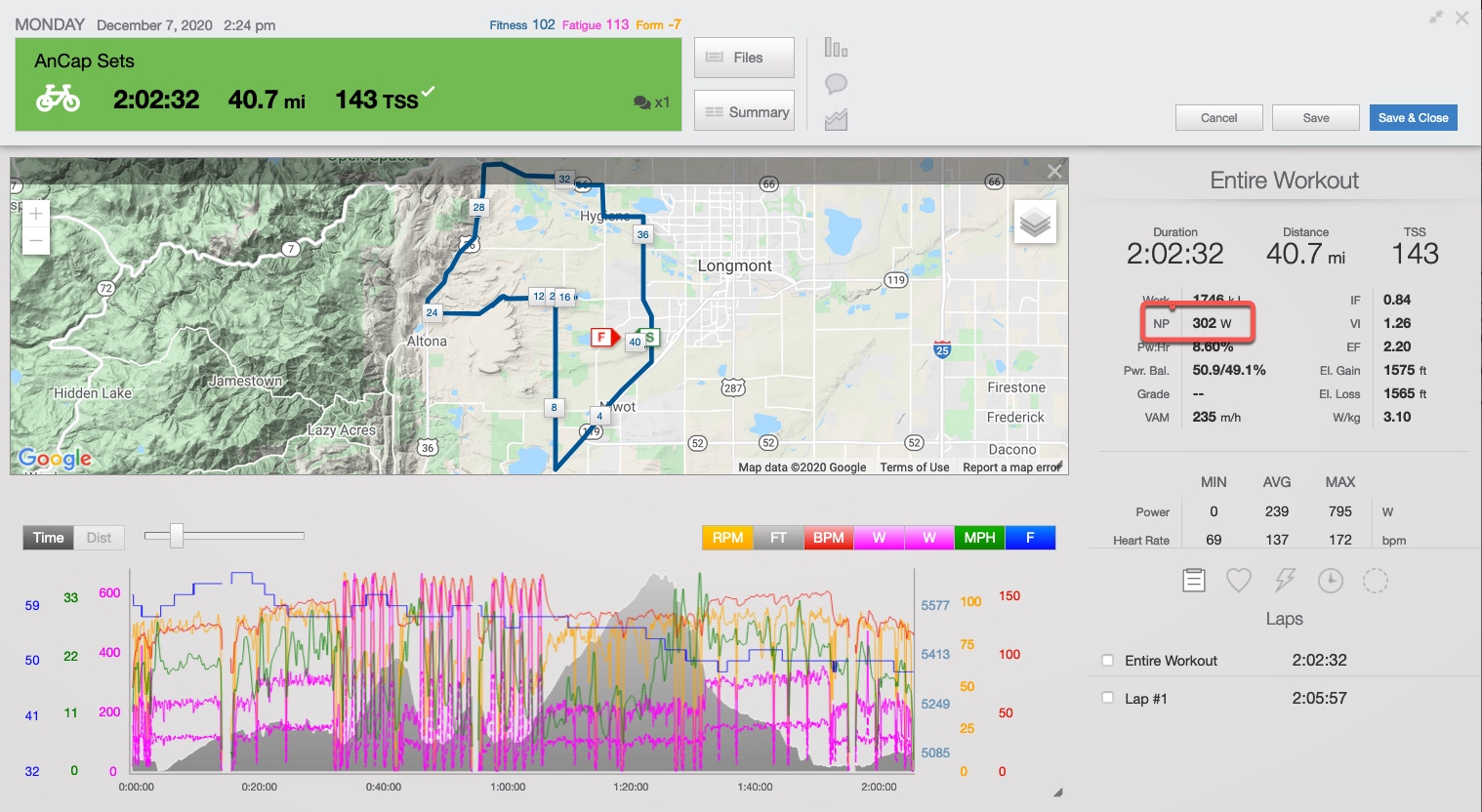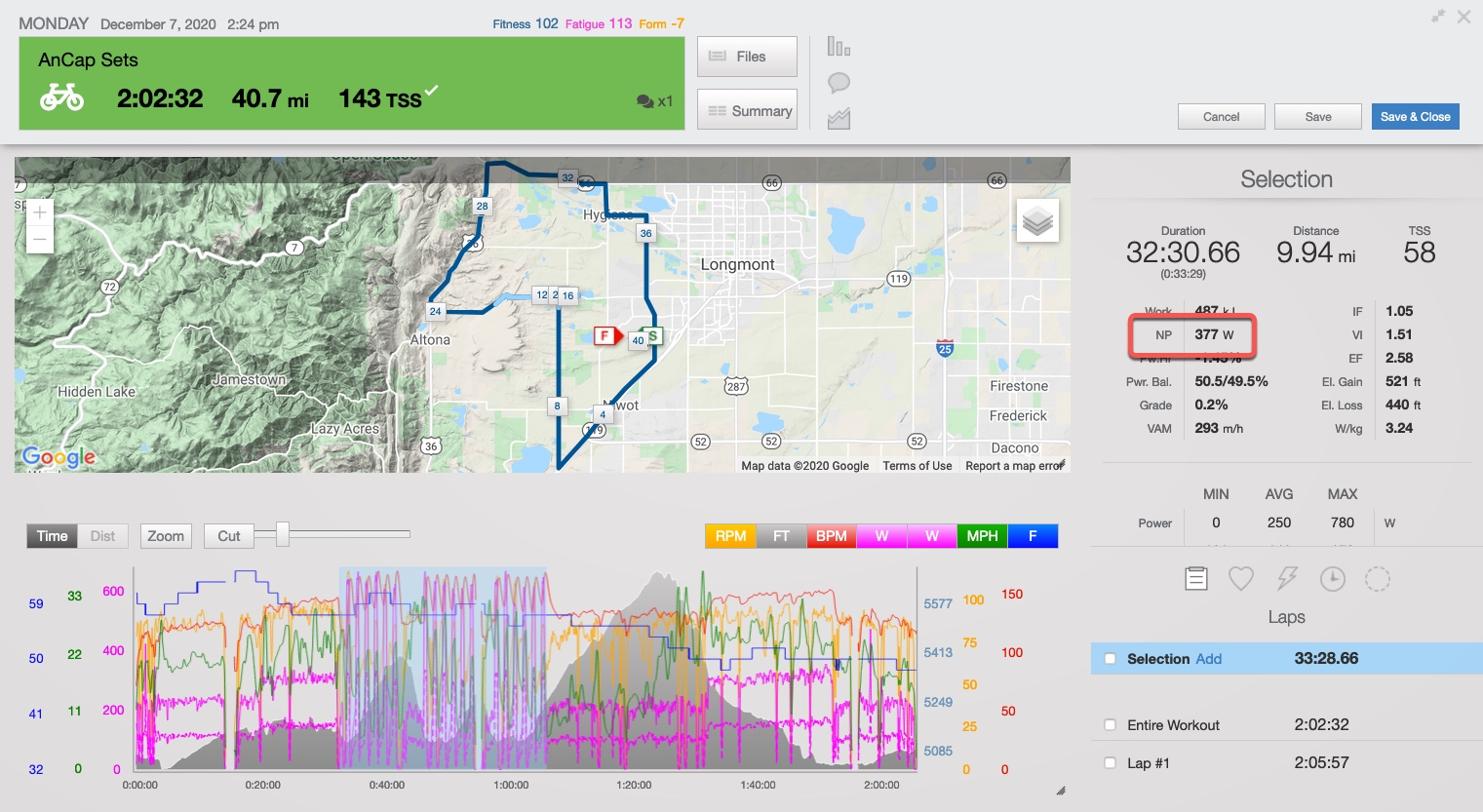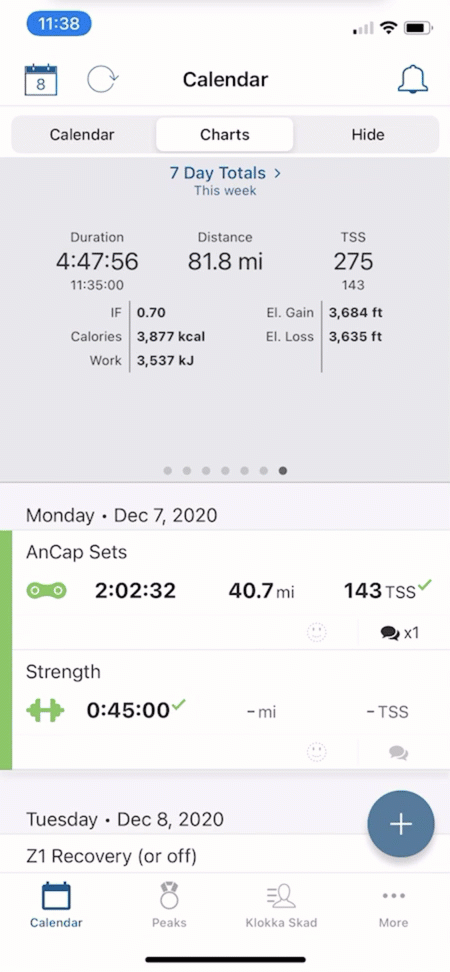# Normalized Power

Normalized Power (NP), together with threshold, allows TrainingPeaks to calculate the Intensity Factor of a workout or selection within a workout.

## What is Normalized Power (NP)

Normalized power (NP) is the adjusted (normalized) average power output for a ride or segment of a ride. Power output on a ride is variable (due to small changes in external power demands e.g. small changes in elevation, small surges in speed, wind, etc) so NP represents the physiological cost of the ride or segment of the ride if that power output had been constant.

## How do I get Normalized Power in TrainingPeaks?

For TrainingPeaks to calculate NP for your ride or segment of the ride we require three things:

1. You need a power meter.
2. A device that records the power channel from your power meter e.g. Garmin, Wahoo, Suunto device.
3. A power threshold that is specific to your completed workout.  Make sure that you have a power threshold set in your account settings.

## Of note

• TrainingPeaks uses Normalized Power to calculate TSS.  TSS = IF^2 (NP/powerFTP) x duration (hours) x 100.
• Ignore Normalized Power calculations for durations under roughly 10 minutes. NP calculations always start with a 30-second rolling average, so shorter NP calculations (< 10 minutes) miss a significant portion of the calculation. e.g. If you select a 3-minute selection in a workout and look at the NP, NP misses almost 20% of the interval before it can calculate an average.
• TrainingPeaks uses the average power from the device file and calculates its Normalized Power. If the average power is higher than the Normalized power highlight a few seconds on the graph in the workout expando and delete it.  The workout edit will prompt TrainingPeaks to calculate the average power which should then produce an average power lower than a normalized power.

## Where can I find my workout's NP?

Workout Expando SummaryWorkout Expando SelectionTrainingPeaks Mobile App